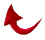IN THE NAME OF GOD MOST GRACIOUS N0ST MERCIFUL

JOURNAL OF SUBMISSION

Volume 1 No. l, March 1993

Editor: Ali R. Fazcly, Ph.D.

Associate Editors: Saied Andalib and Milan Sulc

P. O. Box 80333

Baton Rouge, Louisiana 70808

Introduction

For some time, myself, Mr. Andalib and Mr. Sulc, by God's infinite grace have come across new Quramc mathematical discoveries based on prime numbers and their indices. I have called this Quramc miracle, "ULTIMATE MATHEMATICS". The people in Tucson have been very kind in trying to publish our findings and we are grateful for it. I also tried to publish an article in a scientific journal but so far it has fallen on deaf ears, however, I have not given up. In the mean time, with God's help, the avalanche of these miracles have been so enormous that we decided to start this monthly publication devoted chiefly to the mathematical miracle of the Quran. This gives us a system of referring to our work, and furthermore, we have the responsibility and duty to convey God's miracle to all people. [1 ] In this first issue, we try to give the reader a feeling of how awesome the miracle of Ultimate Mathematics is.

Surely, we have revealed this scripture and surely

we will preserve it, (15:9)

A group theoretical mathematical code based on the "prime" number 19 renders the Quran a unique work of literature never seen in humans' history. This mathematical code involves operations ranging from simple addition (subtraction) and multiplication (division) to sophisticated relations between specific primes and their indices which is beyond our knowledge number theory today. The opening statement which I started this volume with is the foundation upon which this awesome mathematical group has been built.

The mathematical relations which I am presenting here are based on the prime number 19 and how one is able to generate specific crucial numbers in the Quran from this number. Furthermore, the Quran teaches us new mathematics about numbers which was unknown before.

The number 30 is a very important number in the Quran. It can be partitioned into 11 and 19 in which 11 is the 19th number in the Quran. Very important verses in the Quran are labeled 30, like 74:30 "ON IT IS NINETEEN" and 27:30, where the missing Basmallah of chapter 9 is compensated. It is known mathematically that 30 is the largest number where all its smaller relative primes are primes themselves. Note that two numbers are said to be relative primes if they do not possess a common denominator, for example, 3 and 4 are relative primes. What I present below is the mathematics that the Quran teaches us based on the number 19.

In the following table 1 have tabulated all of relative primes to 30 up to 83 with the property that if we add 30 to each of them the sum is also a prime. I have stopped at 83 for several reasons. We know that number 30 is the 23rd number in the Quran and the index of prime number 83 is 23. Furthermore, if we add 83 and 30 we get 113 which is the 30th prime number. Note also that chapter 113 has 23 Arabic words. Also, there is an awesome relation between 42 and 113  and we know that chapter 42 is the 23rd initialed chapter in the Quran. As the reader notes there are 19 such relative primes.

 Table 1 Table of relative primes to 30 COUNT RELATIVE PRIME + 30 = PRIME 1 7 +30=37 2 11 + 30=41 3 13 + 30 = 43 4 17 +30=47 5 23 + 30 = 53 6 29 + 30 = 59 7 31 +30=61 8 37 + 30 = 67 9 41 +30=71 10 43 + 30 = 73 11 49 + 30 = 79 12 53 + 30 = 83 13 59 + 30 = 89 14 67 + 30 = 97 15 71 + 30 = 101 16 73 + 30 = 103 17 77 + 30 = 107 18 79 + 30 = 109 19 83 +30=113

Note that only 17 of these 19 relative primes are primes. The two non-primes are 49 and 77. After adding 30 to each relative prime in the right column of the above table, we have 36 primes. Remember that I stopped at 83. Chapter 36 is the 19th initialed chapter in the Quran and it has 83 verses. On the other hand, Chapter 83 in the Quran has 36 verses.

In Summary, we see that God, the creator of all things has coded the Quran with mathematical relations, yet to be discovered or understood by mankind. This is why chapters are numbered the way they are and possess certain number of verses. No wonder God calls the mathematical miracle of the Quran, one of the greatest miracles. Therefore, let us praise God, creator of all things.

i

 Quran 2:151

 A. R. Fazely, Submitters Perspective, August 1991Return to Table of Contents

/